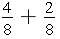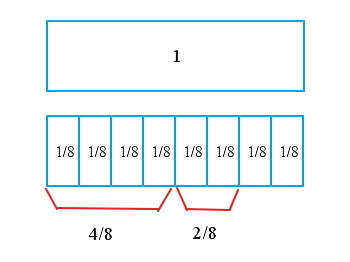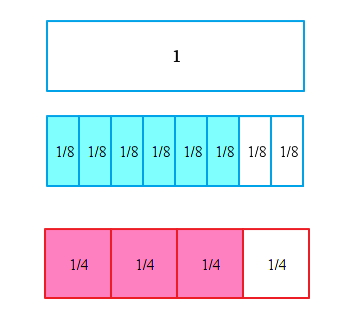Fractions with the same denominators are added by placing the sum of the numerators over the common denominator.  After adding, write the sum in simplest form.

ExampleFirst we add the numerators and the denominators stays the same

Now we write the sum in simplest formWhat If They Do Not Have Common Denominators?

You can find the common denominator: The common denominator is the least common multiple (LCM) of the denominators. You can then rewrite each fraction as an equivalent fraction with that LCM.

Example

Solve:

The multiples for each denominator are

4: 4, 8, 12, 16

3: 3, 6, 9, 12, 15

Therefore the least common multiple (LCM) equals 12, then we can rewrite the fractions using the LCM

Since the denominators are the same we can add numerators and simplify

The sum of a whole number and a fraction is a mixed number.

To add mixed numbers, add the fractions, then add the whole numbers.  Remember to reduce the sum to simplest form.

Example

Solve:

3 + 1 = 4

Remember to reduce the sum to simplest form

Remember that you may need to find the LCM of the fractions in order to add them.

Example

Solve:

Rewrite the fractions using the lowest common denominator

Add the fractions and whole numbers

Write the improper fraction as a mixed number